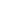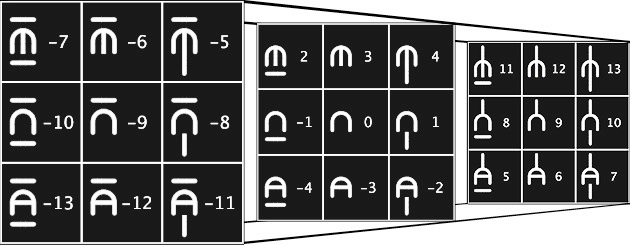This website blog chronicles my exploration into using symbology to represent alternative numerical base systems. My ultimate goal is to uncover and simplify known, and unknown, mathematical processes and connections between these systems. This is a hobby and should be taken as such. Thanks for visiting.

# 014 Balanced Trincubic

Lately, I have been experimenting with assigning Balanced Ternary sub-digits to Trincubic numerals, instead of the aforementioned positive Ternary sub-digits.
``At first glance, it seems very confusing, but it does make the overall system much easier to use. Addition, multiplication, rounding... A person just has to get used to using negative ternary sub-digits.Under Construction

http://en.wikipedia.org/wiki/Balanced_ternary

Unbalanced Trincubic can be converted to Balanced Trincubic by adding ternary sub digits 1111.. with carry, then subtracting 1111... without borrow (the string of 1s must be the same length, so if the result of the addition has more sub digits, subtract nothing from these extra sub digits). For example, 0213 + 1113 = 2023, 2023 − 1113 = 1113(bal) = 710.

``